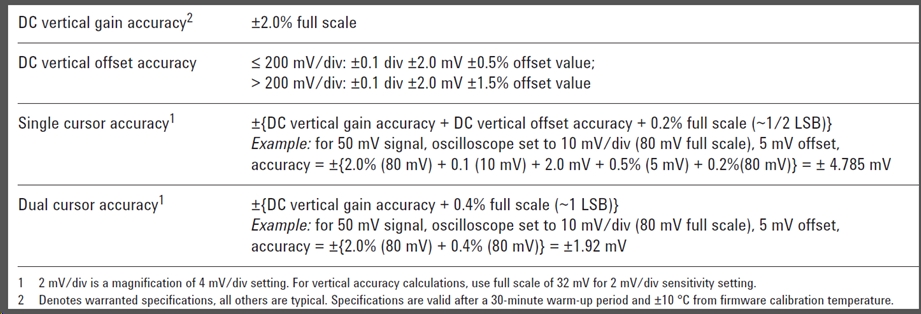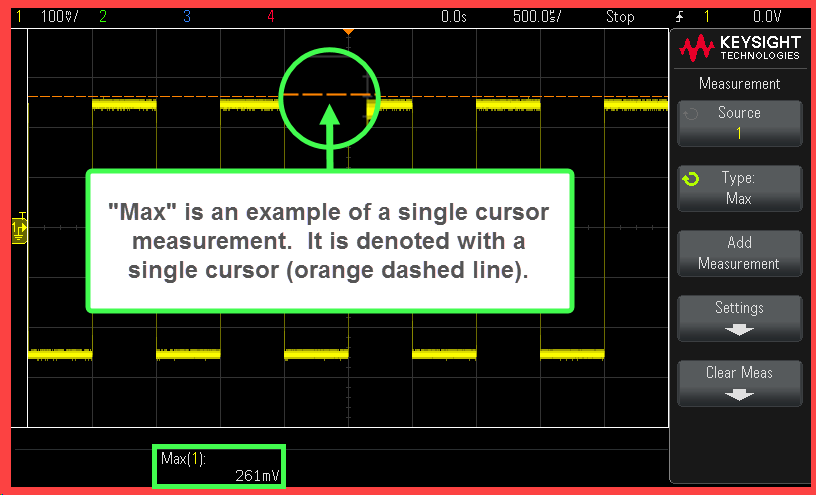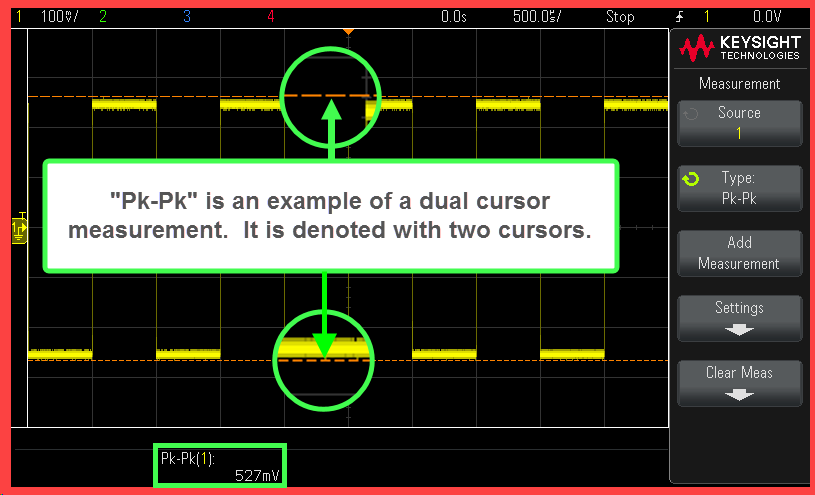save Save

# How is the Voltage Measurement Accuracy for InfiniiVision Oscilloscopes Calculated?

### Summary

Single and dual cursor accuracies are what we really care about. Applies to the following oscilloscope families: • InfiniiVision: DSO5000A, DSO/MSO6000A/L, DSO/MSO7000A/B, EDO/DSOX1000A/G, DSO/MSOX2000A, DSO/MSOX3000A/T, DSO/MSOX4000A, DSO/MSOX6000A, M924xA (PXI), P924xA (USB) • Infiniium: S-Series & MXR families - for the other Infiniium families, the only difference is in DC vertical offset accuracy, but it is very similar.

### Question

How do we calculate the vertical accuracy of InfiniiVision oscilloscope measurements?

If we look in a data sheet for any of the above oscilloscopes, and look in the "Vertical" section (or elsewhere, depending on which data sheet), we will find:

• DC vertical gain accuracy
• DC vertical offset accuracy
• Single cursor accuracy
• Dual cursor accuracy

Here is an excerpt from the InfiniiVision 5000A family data sheet:What are these four terms, what do they mean, and how do we use them?

Before we begin, a few things to keep in mind:

• The proper word for "accuracy" is really "uncertainty", meaning "We know it is somewhere within this range, but we don't really know where."
• All these formulae and sample calculations are for the worst-case, systematic uncertainty.  You can think of this as the maximum, possible, repeatable, measurement error.
• Systematic means that if we make the measurements repeatedly under the same measurement conditions, the error will always be the same.
• Worst-case means we add the terms linearly, we do not root-sum-square (RSS) them. RSS assumes that the uncertainties cancel out partially. That can be acceptable for random uncertainties, but should never be used for cascading systematic uncertainties.
• The uncertainty results are only valid for proper oscilloscope setup and usage.  In many cases, if the signal exceeds the channel's dynamic range (usually this means goes off-screen vertically, but not always), the results will be invalid and corrupt.
• Each of the scopes in these families has its own specifications and caveats, but follow the same general formulae.  Please refer to the relevant data sheets.

DC vertical gain accuracy

This term represents how well the oscilloscope's input attenuation and amplification system works.  You can think of it as the accuracy between any two DC levels on a single channel. This is used as part of the single and dual cursor formulae. Points to note:

• It is given as a percentage of full scale.
• To find full scale,  multiply the vertical scale setting by 8 (these scopes have 8 divisions).
• Example: vertical scale = 1V/div; Full scale = 8 divisions * 1 V/div = 8V
• At the more sensitive scales, software magnification may be used, and full scale may be larger than the scale implies.  This is always denoted in the data sheets.
• This specification is warranted on all Keysight InfiniiVision and Infiniium oscilloscopes.

DC vertical offset accuracy

Oscilloscope channel offset is used to account for a DC level on a signal, so that a signal can be brought onscreen without using too-large a vertical scale (Volts/division setting). Offset accuracy is thus how well the oscilloscope moves the signal up and down the screen. This is used as part of the single cursor formula.

There are a several points to note about the offset accuracy.

• It is given in the vertical units of the channel, typically voltage.
• There are two accuracy ranges, one for up to and including 200 mV/div, and one for above 200 mV/div.  This can be different for different oscilloscope families.
• There are 3 terms in the offset accuracy:

Example ±(0.1 div + 2.0 mV + 0.5% offset value)

• The first term, ±0.1 div, is a zero error, it never goes away.  It dominates the offset accuracy at larger vertical scales.
• The second term, ±2.0 mV, is another zero error that never goes away.  It dominates the accuracy at smaller vertical scales. If you are using a probe, multiply this by the probe attenuation factor.
• Example: using a 10:1 voltage probe, it is now 20 mV
• Example: using a 10:1 current probe (0.1 V/A) gives 2 mV * (1A/100 mV) = 20 mA
• The third term, ±0.5% offset value, is the offset gain accuracy.  If no offset is applied, this term vanishes.
• This is a warranted specification for Infiniium oscilloscopes, but not for InfiniiVision oscilloscopes.

Single cursor accuracy

This can be interpreted in two meaningful ways.  It can be interpreted as:

• Saved data: The vertical accuracy, relative to ground, of any given sample point on a single channel in saved data, e.g. waveform data saved to a USB stick.
• Measurement result: The vertical accuracy of a cursor, whether user-placed or put there by an oscilloscope measurement.  In other words, this is the accuracy of a measurement that uses only one cursor.
• Note that how well a measurement cursor is applied to a waveform depends on scope setup, as well as the measurement algorithm; this does not account for that.
• An example single cursor measurement is Max, i.e. Max Voltage.

There are 3 terms in single cursor accuracy:

• DC vertical gain accuracy
• DC vertical offset accuracy
• The resolution term: 0.2% full scale (~1/2 LSB)
•
• LSB means Least Significant Bit.  The InfiniiVision oscilloscopes are 8-bit oscilloscopes, while the S-series and MXR Infiniium oscilloscopes are 10-bits. The ADCs mapped from the top of the display to the bottom (full scale).
• An LSB is calculated as 1/2^(number of Bits). 1/2 LSB on an 8-bit ADC is 1/2 * 1/2^8 = 1/2 * 1/256 = 1/2 * 0.0039 = 0.00195 = ~2% full scale
• A typical ADC "samples" a signal and puts it at the middle of the closest LSB. The meaning here is that the real signal could be right at the top or bottom of this LSB, so it could be off by as much as 1/2 LSB.
• Average and High Resolution acquisition modes can be used to increase resolution up to 12 bits.  In those scenarios, this term should be recalculated accordingly. On the MXR, the High Resolution mode can be used to 16 bits.

If the ground position is on screen, it is acceptable to short the input (including a probe), measure the zero error, and subtract it from the resulting waveform or measurements, and ignore the two zero error terms in the DC vertical offset accuracy. Measure this at the final settings to be used with the Average - Full Screen measurement, in average acquisition mode.  Note that that the zero error can drift with temperature, so you may want to see how stable it is in your measurement environment. If the ground position is offscreen, this cannot be measured, and thus cannot be removed from the results.Dual cursor accuracy

This represents the vertical accuracy between two cursors on a single channel, whether put there by a user or by a measurement.

• An example dual cursor measurement is Peak-to-Peak (Pk-Pk).
• Note that there is no offset term - on a single channel, the offset accuracy is the same for each channel.
•
• LSB means Least Significant Bit.  The InfiniiVision oscilloscopes are 8-bit oscilloscopes, while the S-series and MXR Infiniium oscilloscopes are 10-bits. The ADCs mapped from the top of the display to the bottom (full scale).
• An LSB is calculated as 1/2^(number of Bits). 1/2 LSB on an 8-bit ADC is 1/2 * 1/2^8 = 1/2 * 1/256 = 1/2 * 0.0039 = 0.00195 = ~2% full scale
• A typical ADC "samples" a signal and puts it at the middle of the closest LSB. The meaning here is that the real signal could be right at the top or bottom of this LSB, so it could be off by as much as 1/2 LSB.
• Average and High Resolution acquisition modes can be used to increase resolution up to 12 bits.  In those scenarios, this term should be recalculated accordingly. On the MXR, the High Resolution mode can be used to 16 bits.
• If you are making vertical measurements between two channels, use two single cursor formulae and add the accuracy results (the accuracy gets worse).Conclusions on Meanings of Terms

• While DC vertical gain accuracy and offset vertical gain accuracy are important for understanding the DC vertical accuracy of an oscilloscope, what really matters for understanding the quality of a result are the single and dual cursor vertical accuracies.
• Single cursor accuracy represents the accuracy of saved waveform data and single cursor measurements, such as Max Voltage.
• Dual cursor accuracy represents the accuracy between two cursors on the same channel, typically used for measurements such as Peak-to-Peak Voltage
• If you are making vertical measurements between two channels, use two single cursor formulae and add the accuracy results (the accuracy gets worse).
• The accuracy is solely a result of the oscilloscope and the settings used.
• Remember these are worst case, systematic uncertainties.
• While a user may be tempted to fiddle with settings to minimize the systematic uncertainty for single cursor results, this can negatively impact the random uncertainty (noise) in a much more significant way.  Specifically, trading a smaller vertical scale for a larger one (worse DC gain accuracy) to use less offset, which often is the largest contribution to the uncertainty, will result in more random noise, and thus a larger standard deviation.
• These formally apply only to DC results.  For AC accuracy, go here.

Example Calculations

Here we will use the above specifications for the 5000A-Series.  We will assume the following settings:

• Vertical scale: 1 V/div
• Offset: 10V
• Average acquisition mode, 256 averages, which gives 12 bits of resolution

DC vertical gain accuracy calculation

Formula

DC vertical gain accuracy = 2% full scale

Calculation

Full scale = 8 divisions * 1 V/div = 8 V

DC vertical gain accuracy = 2% of 8 V = 160 mV

DC vertical offset accuracy calculation

Formula

≤ 200 mV/div: ±(0.1 div + 2.0 mV + 0.5% offset value);

> 200 mV/div: ±(0.1 div + 2.0 mV + 1.5% offset value)

Because the oscilloscope is set to 1 V/div, we use the second formula

Calculation

DC vertical offset accuracy = ±( {0.1 div * 1 V/div} + 2.0 mV + {1.5% offset value * 10 V})

= ±(100 mV + 2.0 mV + 150 mV)

= ±252 mV

Single cursor accuracy calculation

Formula

Single cursor accuracy = ±(DC vertical gain accuracy + DC vertical offset accuracy + 1/2 LSB)

1 LSB = 1/2^(number of bits) * full scale

Calculation

1 LSB = 1/2^12 * 8 V = (1/4096) * 8 V= 0.000244 * 8 V = 1.95 mV

Single cursor accuracy = ±(160 mV + 252 mV + 1/2 * 1.95 mV) = 412.975 mV = ~413 mV

Dual cursor accuracy calculation

Formula

Dual cursor accuracy = ±(DC vertical gain accuracy + 1 LSB)

Calculation

Dual cursor accuracy = ±(160 mV  +  1.95 mV) = 161.95 mV = ~162 mV